SSC CGL Previous Year Questions: Time and Work (Pipe & Cisterns) - 1

# SSC CGL Previous Year Questions: Time and Work (Pipe & Cisterns) - 1 - SSC CGL

Test Description

## 20 Questions MCQ Test SSC CGL (Tier - 1) - Previous Year Papers (Topic Wise) - SSC CGL Previous Year Questions: Time and Work (Pipe & Cisterns) - 1

SSC CGL Previous Year Questions: Time and Work (Pipe & Cisterns) - 1 for SSC CGL 2023 is part of SSC CGL (Tier - 1) - Previous Year Papers (Topic Wise) preparation. The SSC CGL Previous Year Questions: Time and Work (Pipe & Cisterns) - 1 questions and answers have been prepared according to the SSC CGL exam syllabus.The SSC CGL Previous Year Questions: Time and Work (Pipe & Cisterns) - 1 MCQs are made for SSC CGL 2023 Exam. Find important definitions, questions, notes, meanings, examples, exercises, MCQs and online tests for SSC CGL Previous Year Questions: Time and Work (Pipe & Cisterns) - 1 below.
Solutions of SSC CGL Previous Year Questions: Time and Work (Pipe & Cisterns) - 1 questions in English are available as part of our SSC CGL (Tier - 1) - Previous Year Papers (Topic Wise) for SSC CGL & SSC CGL Previous Year Questions: Time and Work (Pipe & Cisterns) - 1 solutions in Hindi for SSC CGL (Tier - 1) - Previous Year Papers (Topic Wise) course. Download more important topics, notes, lectures and mock test series for SSC CGL Exam by signing up for free. Attempt SSC CGL Previous Year Questions: Time and Work (Pipe & Cisterns) - 1 | 20 questions in 20 minutes | Mock test for SSC CGL preparation | Free important questions MCQ to study SSC CGL (Tier - 1) - Previous Year Papers (Topic Wise) for SSC CGL Exam | Download free PDF with solutions
 1 Crore+ students have signed up on EduRev. Have you?
SSC CGL Previous Year Questions: Time and Work (Pipe & Cisterns) - 1 - Question 1

### Vijay alone can complete a work in 50 days. How much part of the work will be completed in ten days?    (SSC MTS 2018)

Detailed Solution for SSC CGL Previous Year Questions: Time and Work (Pipe & Cisterns) - 1 - Question 1

Vijay can do a work alone in 50 days
Work = Efficiency × Time
Hence 1 unit work completed in → 50 days
or 50 days → 1 work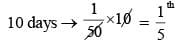Hence 1th / 5 part of the work will be completed in 10 days.

SSC CGL Previous Year Questions: Time and Work (Pipe & Cisterns) - 1 - Question 2

### N and K together can complete a work in 240 days. K and G together can complete the same work in 72 days and N and G together can complete the same work in 80 days. In how many days K alone can complete the same work?    (SSC MTS 2018)

Detailed Solution for SSC CGL Previous Year Questions: Time and Work (Pipe & Cisterns) - 1 - Question 2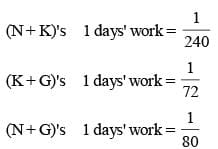On adding, 2(N + K + G)'s 1 days work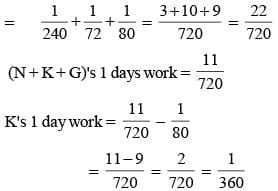Hence, K alone can complete whole work in 360 days.

SSC CGL Previous Year Questions: Time and Work (Pipe & Cisterns) - 1 - Question 3

### The efficiencies of A, B and C are in the ratio of 5 : 3 : 2. Working together, they can complete a task in 21 hours. In how many hours will B alone complete 40% of that task?    (SSC CGL-2018)

Detailed Solution for SSC CGL Previous Year Questions: Time and Work (Pipe & Cisterns) - 1 - Question 3

work done by A, B and C in one day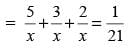x = 10 x 21
Time required to complete 40% work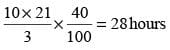SSC CGL Previous Year Questions: Time and Work (Pipe & Cisterns) - 1 - Question 4

A is 40% more efficient than B and C is 20% less efficient than B. Working together, they can finish a work is 5 days. In how many days will A alone complete 70% of that work?    (SSC CGL-2018)

Detailed Solution for SSC CGL Previous Year Questions: Time and Work (Pipe & Cisterns) - 1 - Question 4

Let B alone can finish the whole work in x days
Then A alone can finish the same work in x/1.4 days
and C alone can finish the same work in x/0.8 days.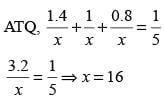Time taken by A to finish 70% of work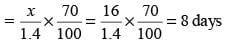SSC CGL Previous Year Questions: Time and Work (Pipe & Cisterns) - 1 - Question 5

It is given that men are twice as efficient than women in respect of doing a work. If three men and two women can complete the work in 2 days, then in how many days can a women working alone complete the work?    (SSC CHSL-2018)

Detailed Solution for SSC CGL Previous Year Questions: Time and Work (Pipe & Cisterns) - 1 - Question 5

1 man = 2 women
work done by 3 men and 2 women in 2 days = 1.
work done by 6 women +2 women in 2 days = 1.
work done by 8 women in 1 day = 1/2.
work done by 1 woman in 1 day = 1/16.
Hence, work is completed by 1 woman in 16 days.

SSC CGL Previous Year Questions: Time and Work (Pipe & Cisterns) - 1 - Question 6

Pipes A and B can fill a tank in 6 hours and 8 hours respectively and pipe C can empty’ the full tank in 12 hours.
All three pipes are opened together, but pipe A is closed after 3 hours. In how many hours will the remaining part of the tank be filled?    (SSC Sub. Ins. 2018)

Detailed Solution for SSC CGL Previous Year Questions: Time and Work (Pipe & Cisterns) - 1 - Question 6

Portion of the tank filled in one hour when all the three pipes open together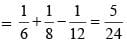Portion of tank filled in 3 hours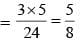Remaining empty tank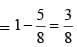Now, when pipe A is closed, then, portion of tank filled by B and C in 1 hour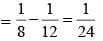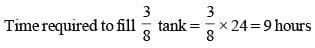SSC CGL Previous Year Questions: Time and Work (Pipe & Cisterns) - 1 - Question 7

36 persons working 8 hours a day can do 3 units of work in 12 days. How many persons are required to do 5 units of that work in 16 days, if they work for 6 hours a day?    (SSC Sub. Ins. 2018)

Detailed Solution for SSC CGL Previous Year Questions: Time and Work (Pipe & Cisterns) - 1 - Question 7

36 persons do 3 units in 12 days working 8 hours a day
∴ 36 persons do 5 units in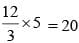days working
8 hours a day Now, when then work for 6 hours a day, then number of worker required to finish the work in 16 days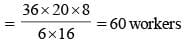SSC CGL Previous Year Questions: Time and Work (Pipe & Cisterns) - 1 - Question 8

Two pipes A and B can fill an empty Tank in 8 hours and 12 hours respectively. They are opened alternately for 1 hour each, starting with pipe A first. In how many hours will the empty tank be filled?    (SSC Sub. Ins. 2018)

Detailed Solution for SSC CGL Previous Year Questions: Time and Work (Pipe & Cisterns) - 1 - Question 8

In first two hours portion of the tank filled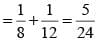Portion of tank filled in 8 hours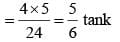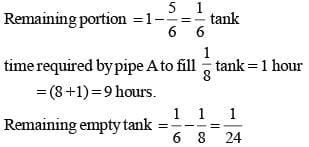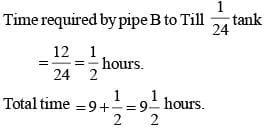SSC CGL Previous Year Questions: Time and Work (Pipe & Cisterns) - 1 - Question 9

The efficiency of A, B and C are in the ratio 5 : 6 : 9. Working together, they can complete a work in 18 days. In how many days can B alone complete 25% of that work?    (SSC Sub. Ins. 2018)

Detailed Solution for SSC CGL Previous Year Questions: Time and Work (Pipe & Cisterns) - 1 - Question 9

Ratio of efficiency of A : B : C = 5 : 6 : 9.
Ratio of time taken by A, B and C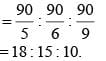Now, from question, together they finish the work in 18 days.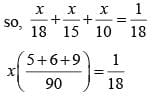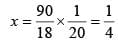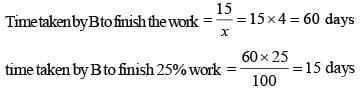SSC CGL Previous Year Questions: Time and Work (Pipe & Cisterns) - 1 - Question 10

Two inlet pipes can fill a cistern in 10 and 12 hours respectively and an outlet pipe can empty 80 gallons of water per hour. All the three pipes working together can fill the empty cistern in 20 hours. What is the capacity (in gallons) of the tank?    (SSC Sub. Ins. 2017)

Detailed Solution for SSC CGL Previous Year Questions: Time and Work (Pipe & Cisterns) - 1 - Question 10

Part filled by first pipe in 1 hour = 1/10
Part filled by second pipe in 1 hour = 1/12
Suppose, the waste pipe can empty the full tank in x hours.
Then, part emptied by waste pipe in 1 hour = 1/x
All the three pipes can fill the tank in 20 hours i.e. part filled by all three pipes in hour = 1/20
Now,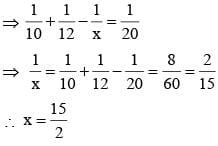i.e, the waste pipe can empty the full tank in 15/2 hours
Given that the waste pipe can empty 80 gallons per hour.
Therefore, in 15/2 hours, it can empty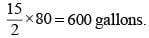SSC CGL Previous Year Questions: Time and Work (Pipe & Cisterns) - 1 - Question 11

P is four times as efficient as Q.P can complete a work in 45 days less than Q. If both of them work together, then in how many days the work will be completed?    (SSC Sub. Ins. 2017)

Detailed Solution for SSC CGL Previous Year Questions: Time and Work (Pipe & Cisterns) - 1 - Question 11

According to question,
If P can complete a work in 1 day,  Q can complete the same work in 4 days.
Hence, if the difference is 3 days, Q can complete the work in 4 days
⇒ If the difference is 45 days, Q can  complete the work in 60 days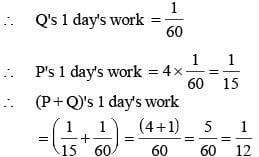∴ P and Q together can do work in 12 days.

SSC CGL Previous Year Questions: Time and Work (Pipe & Cisterns) - 1 - Question 12

A certain number of men complete a piece of work in 60 days. If there were 8 men more, the work can be finished in 10 days less. The number of men originally is:    (SSC MTS 2017)

Detailed Solution for SSC CGL Previous Year Questions: Time and Work (Pipe & Cisterns) - 1 - Question 12

Here,
M1 = x, D1 = 60 days
M2 = (x + 8), D2 = 50 days
Now,
M1D1 = M2D2
⇒ x × 60 = (x + 8) × 50
⇒ 60x = 50x + 400
⇒ 10x = 400
⇒ x = 40
∴ The number of men originally = 40.

SSC CGL Previous Year Questions: Time and Work (Pipe & Cisterns) - 1 - Question 13

A can do a piece of work in 5 days and B in 4 days. How long will they take to do the same work when working together?    (SSC MTS 2017)

Detailed Solution for SSC CGL Previous Year Questions: Time and Work (Pipe & Cisterns) - 1 - Question 13

According to question,
A's 1 day's work = 1/5
B's 1 day's work = 1/4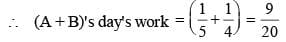∴ A and B can do work together in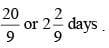SSC CGL Previous Year Questions: Time and Work (Pipe & Cisterns) - 1 - Question 14

A does 80% of a work in 20 days. He then calls in B and they together finish the remaining work in 4 days. How long B alone would take to do the whole work?    (SSC CHSL 2017)

Detailed Solution for SSC CGL Previous Year Questions: Time and Work (Pipe & Cisterns) - 1 - Question 14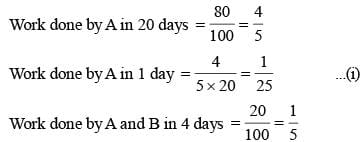(Because remaining 20% is done in 4 days by A and B).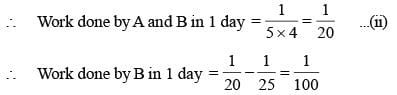∴ B can complete the work in 100 days.

SSC CGL Previous Year Questions: Time and Work (Pipe & Cisterns) - 1 - Question 15

A can do a work in 8 days, B can do the same work in 10 days and C can do the same work in 12 days. If all three of them do the same work together and they are paid ₹ 7400, then what is the share (in ₹ ) of B?    (SSC CGL 2017)

Detailed Solution for SSC CGL Previous Year Questions: Time and Work (Pipe & Cisterns) - 1 - Question 15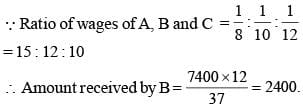SSC CGL Previous Year Questions: Time and Work (Pipe & Cisterns) - 1 - Question 16

A piece of work was finished by A, B, and C together. A and B together finished 60%  of the work and B and C together finished 70% of work. Who among the three is the most efficient?    (SSC CGL 2017)

Detailed Solution for SSC CGL Previous Year Questions: Time and Work (Pipe & Cisterns) - 1 - Question 16

According to question,
A + B = 60%  and B + C = 70%
∴ (A + B) + (B + C) – (A + B + C) = B
(60 + 70 – 100) = 30
∴ B = 30%
A = 30%  and C = 40%
Hence, C is most efficient.

SSC CGL Previous Year Questions: Time and Work (Pipe & Cisterns) - 1 - Question 17

Raman can do a work in 5 days, Jatin can do the same work in 7 days and Sachin can do the same work in 9 days. If they do the same work together and they are paid ₹ 2860, then what is the share (in ₹) of Raman?    (SSC CGL 2017)

Detailed Solution for SSC CGL Previous Year Questions: Time and Work (Pipe & Cisterns) - 1 - Question 17

Raman's 1 day's work = 1/5
Jatin's 1 day's work = 1/7
Sachin's 1 day's work = 1/9
∴ Ratio of their wages = 63 : 45 : 35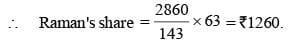SSC CGL Previous Year Questions: Time and Work (Pipe & Cisterns) - 1 - Question 18

A, B and C can complete a work in 20, 24 and 30 days respectively. All three of them starts together but after 4 days A leaves the job and B left the job 6 days before the work was completed. C completed the remaining work alone. In how many days was the total work completed?    (SSC CGL 2017)

Detailed Solution for SSC CGL Previous Year Questions: Time and Work (Pipe & Cisterns) - 1 - Question 18

Suppose, the work was finished in x days. Then, A's 4 day's work + B's (x – 6) day's work + C's x day's work = 1SSC CGL Previous Year Questions: Time and Work (Pipe & Cisterns) - 1 - Question 19

If 4 men and 6 women can complete a work in 8 days, while 3 men and 7 women can complete it in 10 days, then 10 women complete it in    (SSC Sub. Ins. 2016)

Detailed Solution for SSC CGL Previous Year Questions: Time and Work (Pipe & Cisterns) - 1 - Question 19

Let 1 man's 1 day's work = x and 1 woman's 1 day's work = y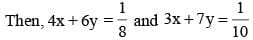Solving two equations,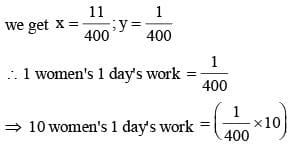Hence, 10 women will complete the work in 40 days.

SSC CGL Previous Year Questions: Time and Work (Pipe & Cisterns) - 1 - Question 20

A and B can separately finish a piece of work in 20 days and 15 days respectively. They worked together for 6 days, after which B was replaced by C. If the work was finished in next 4 days, then the number of days in which C alone could do the work is    (SSC Sub. Ins. 2016)

Detailed Solution for SSC CGL Previous Year Questions: Time and Work (Pipe & Cisterns) - 1 - Question 20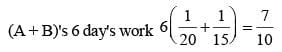(A + C)'s 4 day's work  = 3/10
(A + C)'s 1 day's work  = 3/20
A's 1 day's work = 1/20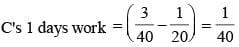Hence C alone can finish the work in 40 days.

## SSC CGL (Tier - 1) - Previous Year Papers (Topic Wise)

250 tests
Information about SSC CGL Previous Year Questions: Time and Work (Pipe & Cisterns) - 1 Page
In this test you can find the Exam questions for SSC CGL Previous Year Questions: Time and Work (Pipe & Cisterns) - 1 solved & explained in the simplest way possible. Besides giving Questions and answers for SSC CGL Previous Year Questions: Time and Work (Pipe & Cisterns) - 1, EduRev gives you an ample number of Online tests for practice

250 tests